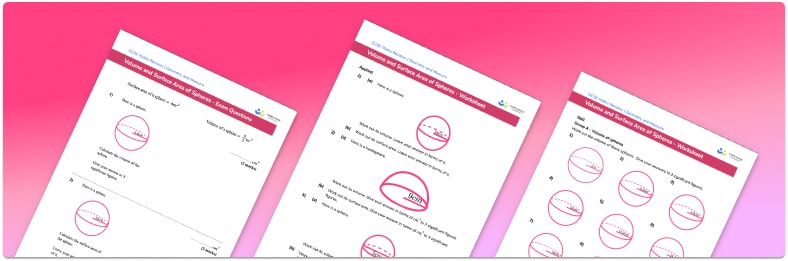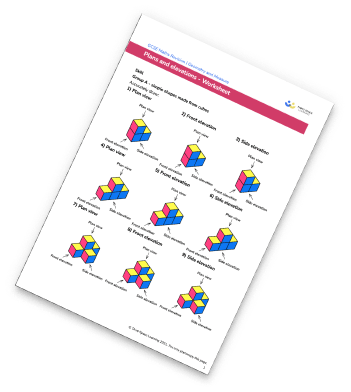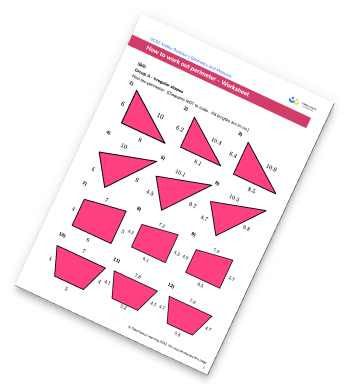# Volume and Surface Area of a Sphere WorksheetHelp your students prepare for their Maths GCSE with this free volume and surface area of a sphere worksheet of 26 questions and answers

• Section 1 of the volume and surface area of a sphere worksheet contains 18 skills-based  volume and surface area of a sphere questions, in 3 groups to support differentiation
• Section 2 contains 5 applied volume and surface area of a sphere questions with a mix of worded problems and deeper problem solving questions
• Section 3 contains 3 foundation and higher level GCSE exam style volume and surface area of a sphere questions
• Answers and a mark scheme for all volume and surface area of a sphere questions are provided
• Questions follow variation theory with plenty of opportunities for students to work independently at their own level
• All questions created by fully qualified expert secondary maths teachers
• Suitable for GCSE maths revision for AQA, OCR and Edexcel exam boards
• To receive this resource and regular emails with more free resources, blog posts and other Third Space updates, enter your email address and click below.

• This field is for validation purposes and should be left unchanged.

You can unsubscribe at any time (each email we send will contain an easy way to unsubscribe). To find out more about how we use your data, see our privacy policy.

### Volume and surface area of a sphere at a glance

The volume of a 3D shape is the amount of space enclosed inside it. The surface area is the total area of all of the faces that make up its surface.

To calculate the volume of a sphere, we use the formula 43r3 , where r is the radius of the sphere. For example, if the radius of a sphere is 4cm, we would substitute the value 4 in for r. This can be input into a calculator and the answer rounded to a given number of decimal places or significant figures. When calculating the volume of the sphere, students can be asked to write their answer as a multiple of , requiring them to simplify the expression as far as possible, but to leave in their answer. This can sometimes result in the answer involving integers or fractions.

To calculate the surface area of a sphere, we use the formula 4r2 . For example, for a sphere with radius 4cm as above, we substitute 4 for r in the formula to calculate the surface area of the sphere. Again, students may be asked to leave their answer as a multiple of

These skills may be applied to compound 3D shapes – for example, calculating the surface area of a hemisphere placed on top of a cylinder. In this case, we calculate the curved surface area of the hemisphere (half of the sphere), the curved surface area of the cylinder, and the area of the circle on the base. To find the total surface area, we then add the three amounts together, and finally round to a suitable number of decimal places.

Looking forward, students can then progress to additional geometry worksheets, for example an angles in polygons worksheet or a 3D shapes worksheet.For more teaching and learning support on Geometry our GCSE maths lessons provide step by step support for all GCSE maths concepts.

## Related worksheets

Plans And Elevations WorksheetPerimeter Worksheet## Do you have KS4 students who need more focused attention to succeed at GCSE?There will be students in your class who require individual attention to help them succeed in their maths GCSEs. In a class of 30, it’s not always easy to provide.

Help your students feel confident with exam-style questions and the strategies they’ll need to answer them correctly with our dedicated GCSE maths revision programme.

Lessons are selected to provide support where each student needs it most, and specially-trained GCSE maths tutors adapt the pitch and pace of each lesson. This ensures a personalised revision programme that raises grades and boosts confidence.

Find out more